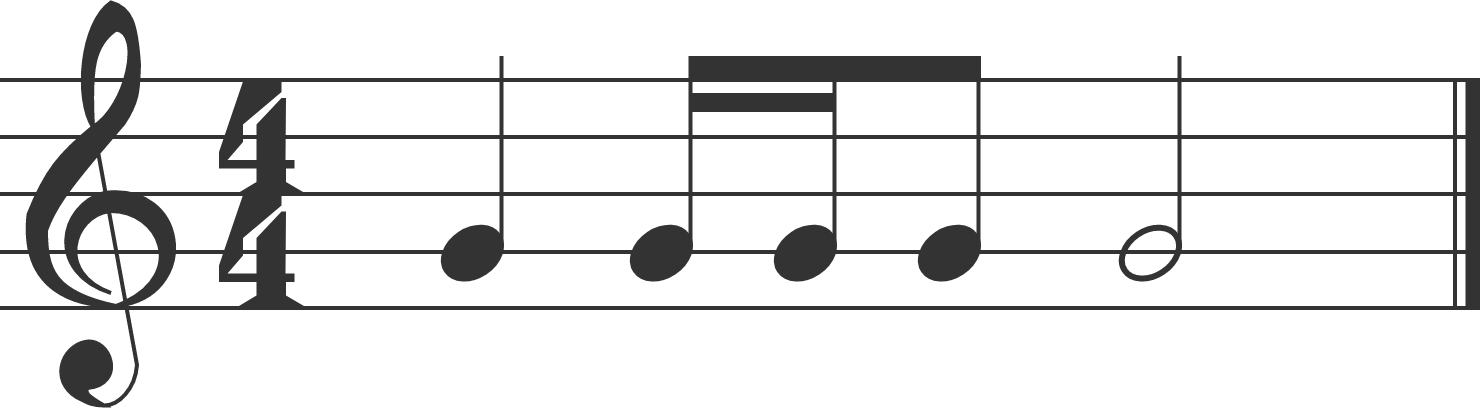# I've Got Rhythm

Probability Level 3

In the common time meter, one bar of music consists of note values equivalent to $4$ quarter notes. Using the note value system, $1$ whole note is equivalent to $2$ half notes, $1$ half note is equivalent to $2$ quarter notes, $1$ quarter note is equivalent to $2$ eighth notes, and $1$ eighth note is equivalent to $2$ sixteenth notes. (For our British friends, a whole note is a semibreve, a half note is a minim, a quarter note is a crotchet, an eighth note is a quaver, and a sixteenth note is a semiquaver.)

An example of a rhythm in one bar of common time meter with $1$ quarter note, $2$ sixteenth notes, $1$ eighth note, and $1$ half note is shown below.Using only whole notes, half notes, quarter notes, eighth notes, and sixteenth notes (with no rests or dotted modifiers), how many unique rhythms can be made in one bar of common time meter?

×